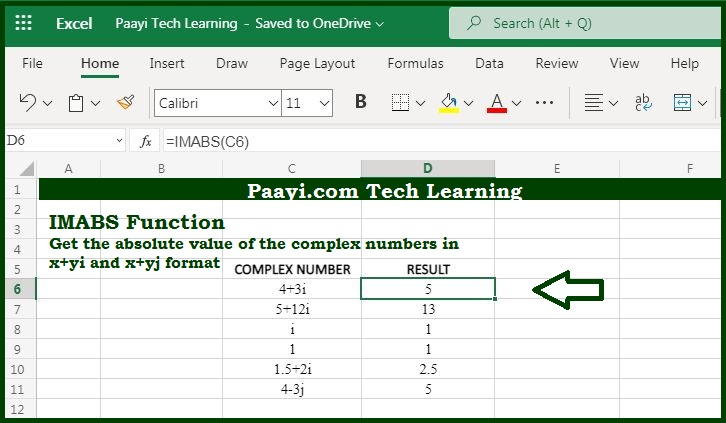# Learn How to Use Microsoft Excel IMABS Function

Written by | 0 Comments | 695 Views

In this article, you will learn how to use the Microsoft Excel IMABS function and its prime function in Microsoft Excel. You will also get to know the Microsoft Excel IMABS function return value and syntax with the help of some examples.

Microsoft Excel IMABS Function

The prime function of the Microsoft Excel IMABS function is to get the absolute value of the complex number. So, with the help of the IMABS function, you can able to return the absolute value of the complex number in the form of  "x + yi" or "x + yj". You can also use the COMPLEX function to create a complex number from real and imaginary parts.

Return Value of IMABS Function

The return value will be the absolute value as a number.

Syntax of IMABS Function

=IMABS(inumber)

Where the arguments:

inumber: It is a complex number.

## How to Use Microsoft Excel IMABS Function?As we know the IMABS function you can able to return the absolute value of the complex number in the form of  "x + yi" or "x + yj". You can also use the COMPLEX function to create a complex number from real and imaginary parts.

It should be noted that only lowercase "i" and "j" are accepted by the IAMBS function and other values will result in a #NUM! error.